Share

# Selina solutions for Concise Mathematics Class 10 ICSE chapter 16 - Loci (Locus and Its Constructions) [Latest edition]

Course
Textbook page

#### Chapters## Chapter 16: Loci (Locus and Its Constructions)

Exercise 16(A)Exercise 16(B)

### Selina solutions for Concise Mathematics Class 10 ICSE Chapter 16 Loci (Locus and Its Constructions) Exercise 16(A) [Pages 237 - 238]

Exercise 16(A) | Q 1 | Page 237

Given: PQ is perpendicular bisector of side AB of the triangle ABC.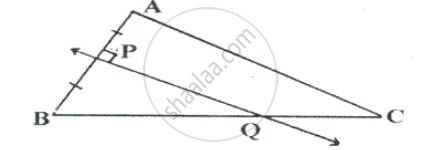Prove: Q is equidistant from A and B.

Exercise 16(A) | Q 2 | Page 237

Given: CP is bisector of angle C of ΔABC.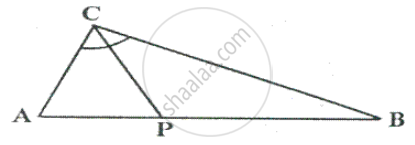Prove: P is equidistant from AC and BC.

Exercise 16(A) | Q 3 | Page 237

Given: AX bisects angle BAC and PQ is perpendicular bisector of AC which meets AX at point Y.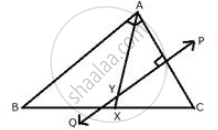Prove: (i) X is equidistant from AB and AC.
(ii) Y is equidistant from A and C.

Exercise 16(A) | Q 4 | Page 237

Construct a triangle ABC, in which AB = 4.2 cm, BC = 6.3 cm and AC = 5cm. Draw perpendicular bisector of BC which meets AC at point D. Prove that D is equidistant from B and C.

Exercise 16(A) | Q 5 | Page 237

In each of the given figures; PA = PB and QA = QB.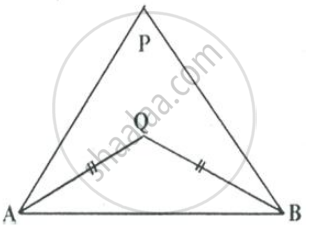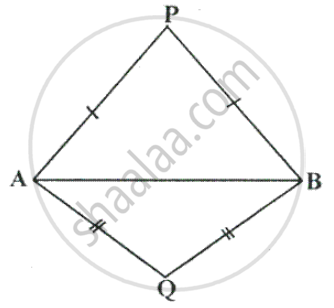Prove, in each case, that PQ (produce, if required) is perpendicular bisector of AB. Hence, state the locus of the points equidistant from two given fixed points.

Exercise 16(A) | Q 6 | Page 237

Construct a right angled triangle PQR, in which ∠Q = 90°, hypotenuse PR = 8 cm and QR = 4.5 cm. Draw bisector of angle PQR and let it meets PR at point t. Prove that T is equidistant from PQ and QR.

Exercise 16(A) | Q 7 | Page 237

Construct a triangle ABC in which angle ABC = 75°, AB= 5cm and BC =6.4cm. Draw perpendicular bisector of side BC and also the bisector of angle ACB. If these bisectors intersect each other at point P; prove that P is equidistant from B and C; and also from AC and BC.

Exercise 16(A) | Q 8 | Page 237

In parallelogram ABCD, side AB is greater than side BC and P is a point in AC such that PB bisects angle B.
Prove that P is equidistant from AB and BC.

Exercise 16(A) | Q 9 | Page 238

In triangle LMN, bisectors of interior angles at L and N intersect each other at point A. prove that:
(i) Point A is equidistant from all the three sides of the triangle.
(ii) AM bisects angle LMN.

Exercise 16(A) | Q 10 | Page 238

Use ruler and compasses only for this question.
(i) Construct ΔABC, where AB = 3.5 cm, BC = 6cm and ∠ABC = 60°.
(ii) Construct the locus of points inside the triangle which are equidistant from BA and BC.
(iii) Construct the locus of points inside the triangle which are equidistant from B and C.
(iv) Mark the point P which is equidistant from AB, BC and also equidistant from B and C. measure and record the length of PB.

Exercise 16(A) | Q 11.1 | Page 238

The given figure shows a triangle ABC in which AD bisects angle BAC. EG is perpendicular bisector of side AB which intersects AD at point F.
Prove that: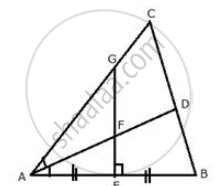F is equidistant from A and B.

Exercise 16(A) | Q 11.2 | Page 238

The given figure shows a triangle ABC in which AD bisects angle BAC. EG is perpendicular bisector of side AB which intersects AD at point F.
Prove that: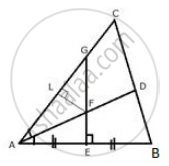(ii) F is equidistant from AB and AC.

Exercise 16(A) | Q 12 | Page 238

The bisectors of ∠B and ∠C of a quadrilateral ABCD intersect each other at point P. Show that P is equidistant from the opposite sides AB and CD.

Exercise 16(A) | Q 13 | Page 238

Draw a line AB = 6 cm. Draw the locus of all the points which are equidistant from A and B.

Exercise 16(A) | Q 14 | Page 238

Draw an angle ABC = 75° Draw the locus of all the points equidistant from AB and BC.

Exercise 16(A) | Q 15 | Page 238

Draw an ∠ABC = 60°, having AB = 4.6 cm and BC = 5cm. Find a point P equidistant from AB and BC; and also equidistant from A and B.

Exercise 16(A) | Q 16 | Page 238

In the figure given below, find a point P on CD equidistant from points A and B.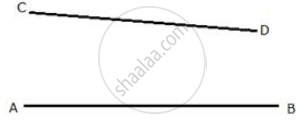Exercise 16(A) | Q 17 | Page 238

In the given triangle ABC, find a point P equidistant from AB and AC; and also equidistant from B and C.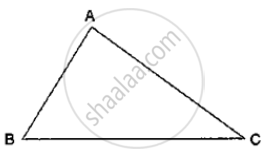Exercise 16(A) | Q 18 | Page 238

Construct a triangle ABC, with AB = 7cm, BC = 8cm and ∠ABC = 60°. Locate by construction the point P such that:
(i) P is equidistant from B and C.
(ii) P is equidistant from AB and BC.
Measure and record the length of PB.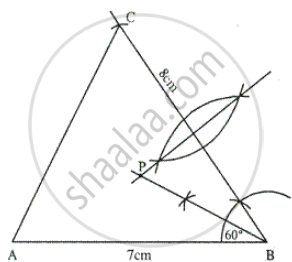Exercise 16(A) | Q 19 | Page 238

On a graph paper, draw the lines x = 3 and y = - 5. Now, on the same graph paper, draw the locus of the point which is equidistant from the given lines.

Exercise 16(A) | Q 20 | Page 238

On a graph paper, draw the line x = 6. Now, on the same graph paper, draw the locus of the point which moves in such a way that its distantce from the given line is always equal to 3 units

### Selina solutions for Concise Mathematics Class 10 ICSE Chapter 16 Loci (Locus and Its Constructions) Exercise 16(B) [Pages 240 - 242]

Exercise 16(B) | Q 1 | Page 240

Describe the locus for questions 1 to 13 given below:
1. The locus of a point at a distant 3 cm from a fixed point.

Exercise 16(B) | Q 2 | Page 240

Describe the locus for questions 1 to 13 given below:

The locus of points at a distance 2cm from a fixed line.

Exercise 16(B) | Q 3 | Page 240

Describe the locus for questions 1 to 13 given below:

The locus of the centre of a wheel of a bicycle going straight along a level road.

Exercise 16(B) | Q 4 | Page 240

Describe the locus for questions 1 to 13 given below:

The locus of the moving end of the minute hand of a clock.

Exercise 16(B) | Q 5 | Page 240

Describe the locus for questions 1 to 13 given below:

The locus of a stone dropped from the top of a tower.

Exercise 16(B) | Q 6 | Page 241

Describe the locus for questions 1 to 13 given below:

The locus of a runner, running round a circular track and always keeping a distance of 1.5 m from the inner edge.

Exercise 16(B) | Q 7 | Page 241

Describe the locus for questions 1 to 13 given below:

The locus of the door handle, as the door opens .

Exercise 16(B) | Q 8 | Page 241

Describe the locus for questions 1 to 13 given below:

The locus of points inside a circle and equidistant from two fixed points on the circumference of the circle.

Exercise 16(B) | Q 9 | Page 241

Describe the locus for questions 1 to 13 given below:

The locus of the centres of all circles passing through two fixed points.

Exercise 16(B) | Q 10 | Page 241

Describe the locus for questions 1 to 13 given below:

The locus of vertices of all isosceles triangles having a common base.

Exercise 16(B) | Q 11 | Page 241

Describe the locus for questions 1 to 13 given below:

The locus of a point in space, which is always at a distance of 4cm from a fixed point.

Exercise 16(B) | Q 12 | Page 241

Describe the locus for questions 1 to 13 given below:

The locus of a point P, so that:
AB^2 = AP^2 + BP^2,
Where A and B are two fixed points.

Exercise 16(B) | Q 13 | Page 241

Describe the locus for questions 1 to 13 given below:

The locus of a point in rhombus ABCD, so that it is equidistant from
(i) AB and BC; (ii) B  and D .

Exercise 16(B) | Q 14 | Page 241

The speed of sound is 332 metres per second. A gun is fired. Describe the locus of all the people on the earth’s surface, who hear the sound exactly one second later.

Exercise 16(B) | Q 15.1 | Page 241

Describe:

The locus of points at distances less than 3cm from a given point.

Exercise 16(B) | Q 15.2 | Page 241

Describe:

The locus of points at distances greater than 4 cm from a given point.

Exercise 16(B) | Q 15.3 | Page 241

Describe:

The locus of points at distances less than or equal to 2.5 cm from a given point.

Exercise 16(B) | Q 15.4 | Page 241

Describe:

The locus of points at distances greater than or equal to 35 mm from a given point.

Exercise 16(B) | Q 15.5 | Page 241

Describe:

The locus of the centres of a given circle which rolls around the outside of a second circle and is always touching it.

Exercise 16(B) | Q 15.6 | Page 241

Describe:

The locus of the centres of all circles that are tangent to both the arms of a given angle.

Exercise 16(B) | Q 15.7 | Page 241

Describe:

The locus of the mod-points of all chords parallel to a given chord of a circle.

Exercise 16(B) | Q 15.8 | Page 241

Describe:

The locus of points within a circle that are equidistant from the end points of a given chord.

Exercise 16(B) | Q 16 | Page 241

Sketch and describe the locus of the vertices of all triangles with a given base and a given altitude.

Exercise 16(B) | Q 17 | Page 241

In the given figure, obtain all the points equidistant from lines m and n; and 2.5 cm from O.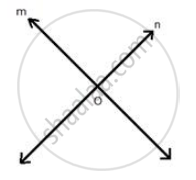Exercise 16(B) | Q 18 | Page 241

A straight line AB is 8cm long. Draw and describe the locus of a point which is:
(i) always 4 cm from the line AB.
(ii) equidistant from A and B.
Mark the two points X and Y, which are 4cm from AB and equidistant from A and B. describe the figure AXBY.

Exercise 16(B) | Q 19 | Page 241

Angle ABC = 60° and BA = BC = 8 cm. The mid points of BA and BC are M and N respectively. Draw and describe the locus of a point which is:
(i) Equidistant from BA and BC.
(ii) 4 cm from M
(iii) 4 cm from N
Mark the point P, which is 4 cm from both M and N, and equidistant from BA and BC. Join MP and NP, and describe the figure BMPN.

Exercise 16(B) | Q 20 | Page 241

Draw a triangle ABC in which AB = 6cm, BC = 4.5 cm and AC = 5cm. Draw and label:
(i) the locus of the centres of all circles which touch AB and AC,
(ii) the locus of the centres of all the circles of radius 2 cm which touch AB.
Hence, construct the circle of radius 2cm which touches AB and AC .

Exercise 16(B) | Q 21 | Page 241

Construct a triangle ABC, having given AB = 4.8 cm, AC = 4cm, and ∠A = 75°.
Find a point P.
(i) Inside the triangle ABC.
(ii) outside the triangle ABC
Equidistant from B and C; and at a distance of 1.2 cm from BC.

Exercise 16(B) | Q 22 | Page 241

Draw an angle ABC = 75°. Find a point P such that P is at a distance of 2 cm from AB and 1.5 cm from BC.

Exercise 16(B) | Q 23 | Page 241

Construct a triangle ABC, with AB = 5.6 cm, AC = BC = 9.2 cm. Find the points equidistant from AB and AC; and also 2 cm from BC. Measure the distance between the two points obtained.

Exercise 16(B) | Q 24 | Page 241

Construct a triangle ABC, with AB = 6 cm, AC = BC = 9cm. Find a point 4 cm from A and equidistant from B and C.

Exercise 16(B) | Q 25 | Page 241

Ruler and compasses may be used in this question. All construction lines and arcs must be clearly shown and be of sufficient length and clarity to permit assessment.
(i) Construct a ΔABC, in which BC = 6cm, AB = 9 cm and angle ABC = 60°.
(ii) Construct the locus of all points inside triangle ABC, which are equidistant from B and C.
(iii) Construct the locus of the vertices of the triangles with BC as base and which are equal in area to triangle ABC.
(iv) Mark the point Q, in your construction, which would make ΔQBC equal in area to ΔABC, and isosceles.
(v) Measure and record the length of CQ.

Exercise 16(B) | Q 26 | Page 241

State the locus of a point in a rhombus ABCD, which is equidistant
(ii) from the vertices A and C.

Exercise 16(B) | Q 27 | Page 241

Use graph paper for this question. Take 2 cm = 1 unit on both the axis.
(i) Plot the points A(1,1), B(5,3) and C(2,7).
(ii) Construct the locus of points equidistant from A and B.
(iii) Construct the locus of points equidistant from AB and AC.
(iv) locate the point P such that PA = PB and P is equidistant from AB and AC.
(v) Measure and record the length PA in cm.

Exercise 16(B) | Q 28 | Page 241

Construct an isosceles triangle ABC such that AB = 6cm, BC = AC = 4cm. Bisect ∠C internally and mark a point P on this bisector such that CP = 5 cm. Find the points Q and R which are 5 cm from P and also 5 cm from the line AB.

Exercise 16(B) | Q 29 | Page 241

Plot the points A(2, 9), B(-1, 3) and C (6, 3) on graph paper. On the same graph paper draw the locus of point A so that the area of ΔABC remains the same as A moves.

Exercise 16(B) | Q 30 | Page 241

Construct a triangle BPC given BC = 5 cm, BP = 4 cm and .

i) complete the rectangle ABCD such that:
a) P is equidistant from AB and BCV
b) P is equidistant from C and D.
ii) Measure and record the length of AB.

Exercise 16(B) | Q 31 | Page 242

Use ruler and compass only for the following question. All construction lines and arcs must be clearly shown.

(i) Construct a ΔABC in which BC = 6.5 cm, ∠ABC = 60°, AB = 5 cm.

(ii) Construct the locus of points at a distance of 3.5 cm from A.

(iii.) Construct the locus of points equidistant from AC and BC.

(iv)Mark 2 points X and Y which are at a distance of 3.5 cm from A and also equidistant from AC and BC. Measure XY.

## Chapter 16: Loci (Locus and Its Constructions)

Exercise 16(A)Exercise 16(B)## Selina solutions for Concise Mathematics Class 10 ICSE chapter 16 - Loci (Locus and Its Constructions)

Selina solutions for Concise Mathematics Class 10 ICSE chapter 16 (Loci (Locus and Its Constructions)) include all questions with solution and detail explanation. This will clear students doubts about any question and improve application skills while preparing for board exams. The detailed, step-by-step solutions will help you understand the concepts better and clear your confusions, if any. Shaalaa.com has the CISCE Concise Mathematics Class 10 ICSE solutions in a manner that help students grasp basic concepts better and faster.

Further, we at Shaalaa.com provide such solutions so that students can prepare for written exams. Selina textbook solutions can be a core help for self-study and acts as a perfect self-help guidance for students.

Concepts covered in Concise Mathematics Class 10 ICSE chapter 16 Loci (Locus and Its Constructions) are Introduction of Loci, Loci Examples, Constructions Under Loci, Theorems Based on Loci.

Using Selina Class 10 solutions Loci (Locus and Its Constructions) exercise by students are an easy way to prepare for the exams, as they involve solutions arranged chapter-wise also page wise. The questions involved in Selina Solutions are important questions that can be asked in the final exam. Maximum students of CISCE Class 10 prefer Selina Textbook Solutions to score more in exam.

Get the free view of chapter 16 Loci (Locus and Its Constructions) Class 10 extra questions for Concise Mathematics Class 10 ICSE and can use Shaalaa.com to keep it handy for your exam preparation